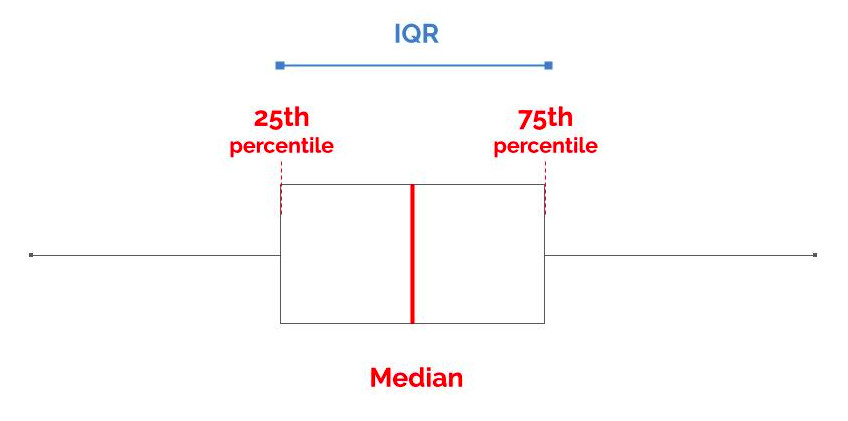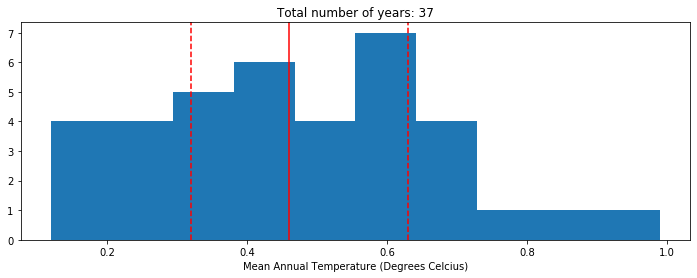# Interquartile Range (IQR) – From Scratch in Python

## Introduction

Pre-requisite: Quartiles, Quantiles and Percentiles

The Interquartile range (IQR) is the difference between the 75th percentile (0.75 quantile) and the 25th percentile (0.25 quantile). The IQR can be used to detect outliers in the data.## Python Practice

import pandas as pd
import numpy as np
import matplotlib.pyplot as plt

%matplotlib inline


### 1 – Dataset

For this tutorial, we will use the global average temperatures from 1980 to 2016. The original dataset can be found on Datahub.io.

df = pd.read_csv("../data/temperature_CO2.csv")
df = df.dropna()
df = df[['year', 'temperature']]
df.head(3)
year temperature2016 0.9912015 0.8722014 0.74

### 2 – Compute the percentiles

To compute the IQR, we need to know which temperature corresponds to:

• the 25th percentile (ie, warmer than 25% of the temperatures in this dataset)
• the 75th percentile (ie, warmer than 75% of the temperatures in this dataset)

To achieve this, first sort your dataset by ascending temperature, and reset the indices.

df = df.sort_values(by='temperature').reset_index(drop=True)
df.head()
year temperature1985 0.1211982 0.1321984 0.1531986 0.1941992 0.23

Then, use a rule of three to find the index of the value corresponding to your percentile rank. Example for the 25th percentile:

$$\textbf{length(data)} -1 \longrightarrow 100^{th} \text{percentile}$$

$$\textbf{length(x)} \longrightarrow 25^{th} \text{percentile}$$

The -1 takes into account the fact that indices start at zero. So

def get_percentile(df, percentile_rank):

# First, sort by ascending temperature, reset the indices
df = df.sort_values(by='temperature').reset_index()

# Rule of three to get the index of the temperature
index = (len(df.index)-1) * percentile_rank / 100.0
index = int(index)

# Return the temperature corresponding to the percentile rank
return df.at[index, 'temperature']

So we see that the 25th percentile is 0.32 degrees Celsius, and the 75th percentile is 0.63 degrees Celsius.

get_percentile(df, 25)
>>> 0.32

get_percentile(df, 75)
>>> 0.63


### 2 – Compute the IQR

Almost done: since the interquartile range (IQR) is the difference between the 75th percentile and the 25th percentile, all we need to do is to subtract both temperature values.

def interquartile_range(df):

p75 = get_percentile(df, 75)  # 75th percentile
p25 = get_percentile(df, 25)  # 75th percentile
iqr = p75 - p25  # Interquartile Range
return iqr
interquartile_range(df)
>>> 0.31


### 3 – Validation

Coding the IQR from scratch is a good way to learn the math behind it, but in real life, you would use a Python library to save time. We can use the iqr() function from scipy.stats to validate our result.

from scipy.stats import iqr

iqr(df['temperature'])
>>> 0.31

### 4 – Visualization

Let’s plot the 25th percentile, the 50th percentile (median) and the 75th percentile of the data.

plt.figure(figsize=(12,4))
plt.hist(df['temperature'])
plt.title("Total number of years: %s" % len(df.index))
plt.xlabel("Mean Annual Temperature (Degrees Celcius)")

# Vertical lines for each percentile of interest
plt.axvline(get_percentile(df, 25), linestyle='--', color='red')
plt.axvline(get_percentile(df, 50), linestyle='-',  color='red')
plt.axvline(get_percentile(df, 75), linestyle='--', color='red')

plt.show()For a fully working Python notebook check my Github.